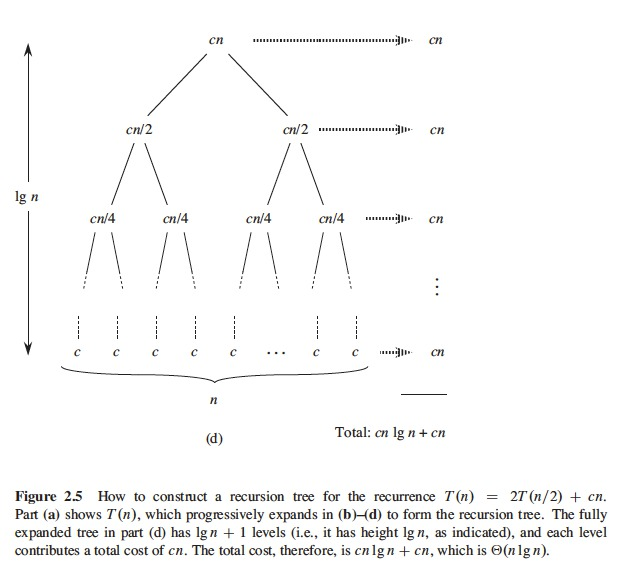July 17, 2019

# Getting Started

• These slides are based on the book:
• Introduction to Algorithms
• Thomas H. Cormen, Charles E. Leiserson, Ronald L. Rivest, and Clifford Stein
• The MIT Press, Third Edition
• ISBN: 978-0-262-03384-8 (hardcover: alk. paper)
• ISBN: 978-0-262-53305-8 (pbk: alk. paper)

# Content & Concepts

• Insertion sort
• Loop Invariant
• Proof Correctness
• Pseudocode Conventions
• Analyzing Algorithms
• Order of Growth
• Efficient Algorithm
• Designing Algorithms
• Incremental Approach
• Divide and Conquer
• Greedy Choice
• Dynamic Programming
• Divide and Conquer
• Recursive Algorithm
• Recurrence
• Merge Sort

# Insertion Sort

• Our first algorithm is Insertion Sort
• Solves the sorting problem
• Input: A sequence of $$n$$ numbers $$\langle a_1, a_2, \dots, a_n \rangle$$.
• Output: A permutation (reordering) $$\langle a'_1, a'_2, \dots, a'_n \rangle$$ of the input sequence such that $$a'_1 \leq a'_2 \dots, \leq a'_n$$.

# Insertion Sort

• We use pseudocode to describe algorithms
• Similar to C, C++, Java, Python, Pascal
• Very descriptive
• Sometimes sentences in English
• We will describe insertion sort with pseudocode

# Insertion Sort

• How does insertion sort work?
• Similar to the way we sort a hand of playing cards
• Start with empty left hand, cards face down on the table
• Take one card at a time and insert it to its correct position in the left hand
• Compare card with cards already in the hand
• From right to left
• We always keep our cards in the left hand sorted

# Insertion Sort

• Analysis of Insertion Sort
• Execution time depends on the input
• Input size
• Is the input partially ordered?
• Input size
• Number of elements in the input
• A vector, the number of elements
• Graphs, number of vertices, number of edges

# Insertion Sort

• Insertion sort with input $$A = \langle 5, 2, 4, 6, 1, 3 \rangle$$
• $$j$$ indicates current card being inserted
• $$A[1 \dots j-1]$$, currently sorted hand
• $$A[j+1 \dots n]$$, cards still to be sorted

# Insertion Sort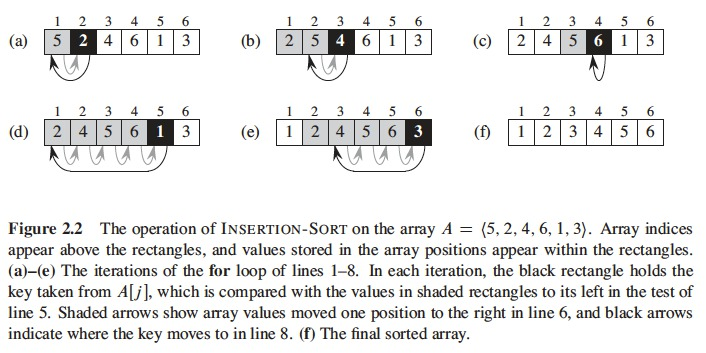# Insertion Sort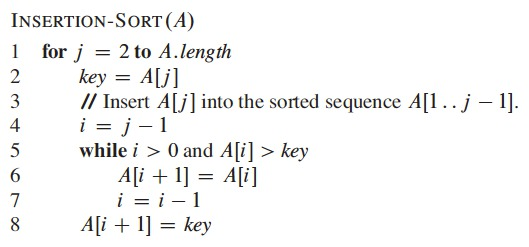# Insertion Sort - Loop Invariant

• Loop invariant
• Property of a program loop
• It holds before, during, and after each iteration of the loop
• We use loop invariants to understand why an algorithm is correct
• We must show three things:
• Initialization: It is true prior to the first iteration of the loop
• Maintenance: If it is true before an iteration of the loop, it remains true before the next iteration
• Termination: When the loop terminates, the invariant gives us a useful property that helps show that the algorithm is correct

# Insertion Sort - Loop Invariant

• Loop invariant for Insertion Sort
• Initialization
• $$j = 2$$, $$A[1, \dots j-1]$$ has only $$A$$
• $$A$$ is sorted (trivially)
• The loop invariant holds prior to the first iteration of the loop

# Insertion Sort - Loop Invariant

• Loop invariant for Insertion Sort
• Maintenance
• The for loop moves $$A[j-1], A[j-2], A[j-3]$$ and so on
• Inserts $$A[j]$$ in the right position
• $$A[1..j]$$ contains the elements originally in $$A[1..j]$$ but in sorted order
• Incrementing $$j$$ for next iteration preserves the loop invariant
• Formally, we should show the loop invariant for the while loop as well

# Insertion Sort - Loop Invariant

• Loop invariant for Insertion Sort
• Termination
• Examine what happens when the loop terminates
• See condition $$j > A.length = n$$
• Each loop iteration increases $$j$$ by 1
• It finishes when we have $$j = n + 1$$
• We have the array $$A[1..n]$$
• The algorithm finishes when all numbers $$A[1..n]$$ have been sorted
• Then, the algorithm is correct

# Pseudocode Conventions

• Indentation shows block structure
• Symbol // indicates remainder of line is a comment
• We pass parameters to a procedure by value
• Procedure receives its own copy

# Analyzing Algorithms

• Analyzing an algorithm
• Predicting the resources that the algorithm requires
• Mostly computational time
• We analyze several candidates and choose the best one (most efficient) for the problem at hand
• Need to know the model of the implementation technology
• We assume a generic one processor
• Random-access machine (RAM), one instruction after the other with no concurrent operations

# Analyzing Algorithms

• We measure running time
• Number of primitive operations (steps) executed
• Need to define the notion of step in a way independent from the computer
• Constant time to execute each pseudocode line
• Add, Multiply, divide, floor, remainder, and so on
• They actually take different time but we treat it as the same and as constant time

# Analyzing Algorithms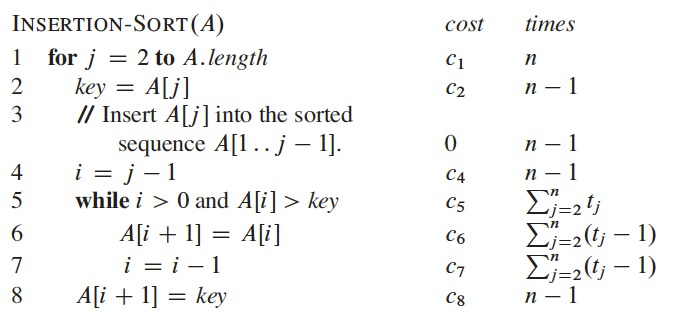# Analyzing Algorithms

• For each $$j = 2, 3, \dots, n$$, $$n = length[A]$$
• $$t_j$$ is the number of executions of the while cycle in line 5 for the value of $$j$$
• Running time is the sum of the times of each line
• $$T(n) = c_1 n + c_2(n - 1) + c_4(n - 1) + c_5 \sum_{j=2}^{n}t_j$$
• $$+ c_6 \sum_{j=2}^{n} (t_j - 1) + c_7 \sum_{j=2}^{n} (t_j -1) + c_8(n - 1)$$.

# Analyzing Algorithms

• Best case
• Array of numbers previously sorted
• Can be expressed as $$an + b$$, a linear function of $$n$$

$$T(n) = c_1 n + c_2 (n-1) + c_4(n-1) + c_5(n-1) + c_8(n-1)$$ $$T(n) = (c_1 + c_2 + c_4 + c_5 + c_8)n - (c_2 + c_4 + c_5 + c_8)$$

# Analyzing Algorithms

• Worst case
• The array has a decreasing sorting
• We compare each element $$A[j]$$ with each element of subarray $$A[1..j-1]$$ and $$t_j = j$$ for $$j = 2, 3, \dots, n$$, where:
• $$\sum_{j=2}^{n} j = \frac{n(n+1)}{2}-1$$.
• $$\sum_{j=2}^{n} (j-1) = \frac{n(n-1)}{2}$$.

# Analyzing Algorithms

• Worst case
• Can be expressed as $$an^2 + bn + c$$, which is a quadratic function

$$T(n) = c_1n + c_2(n-1) + c_4(n-1) + c_5 \left( \frac{n(n+1)}{2} -1 \right)$$ $$+ c_6 \left( \frac{n(n-1)}{2} \right) + c_7 \left( \frac{n(n-1)}{2} \right) + c_8(n-1)$$

$$T(n) = \left( \frac{c_5}{2}+\frac{c_6}{2}+\frac{c_7}{2} \right) n^2$$ $$+ \left(c_1 + c_2 + c_4 + \frac{c_5}{2} - \frac{c_6}{2} - \frac{c_7}{2} + c_8 \right)n$$ $$-(c_2 + c_4 + c_5 + c_8)$$.

# Analyzing Algorithms

• We usually only compute the worst case
• The longest running time for each size of entry $$n$$
• The worst case running time is the upper bound
• We guarantee that the algorithm won’t take longer than that upper bound
• For some algorithms, worst case happens frequently (i.e. DB search)
• Average case is usually as bad as the worst case

# Analyzing Algorithms

• Order of Growth
• We simplify the analysis of algorithms
• We use constants to represent the cost of lines of code
• Another simplification (abstraction)
• We only care about the growing rate or order of growth
• We only consider the leading term in a formula (i.e. $$an^2$$)
• We also ignore constant coefficients
• They are less significant than the growing rate

# Analyzing Algorithms

• Order of Growth
• Worst case for InsertionSort: $$\theta(n^2)$$
• Theta of $$n$$-squared
• We say that an algorithm is more efficient than another one if its worst case running time has a lower order of growth
• There might be inconsistencies for short input sizes but not for large ones

# Designing Algorithms

• Many techniques to design algorithms
• InsertionSort follows an incremental approach
• Other techniques such as
• Divide and conquer

# Designing Algorithms

• Divide and Conquer
• Many algorithms are recursive in their structure
• Recursive algorithms call themselves once or more times to solve similar subproblems
• They follow a divide and conquer approach
• Divide the problem in similar subproblems but smaller
• Solve the problems recursively
• Combine the solutions to create the solution to the original problem

# Designing Algorithms

• Divide and Conquer
• 3 steps in each level of recursion
• Divide the problem in subproblems
• Conquer the problems by recursively solving them, if the problem is trivial (small enough) it solves it directly
• Combine the solutions to obtain the solution to the original problem

# Designing Algorithms

• MergeSort follows the divide-and-conquer paradigm
• Divides the sequence of $$n$$-elements to sort in 2 subsequences of $$n/2$$ elements each
• Sorts the 2 subsequences recursively using MergeSort
• Combines, merges the 2 sorted subsequences to produce the sorted solution

# Designing Algorithms

• Merge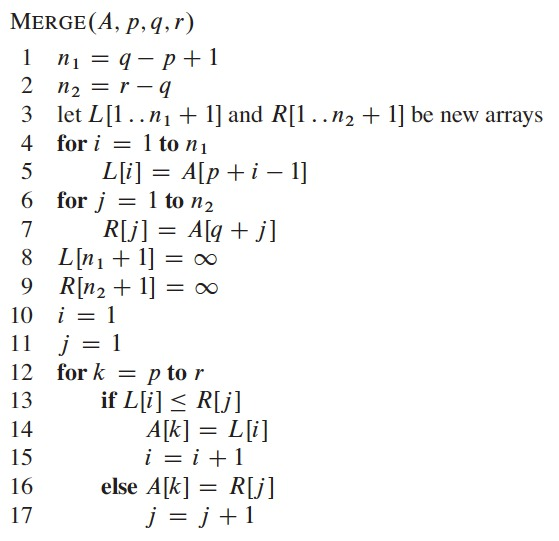# Designing Algorithms

• MergeSort:
• Initially $$A$$ is the input array, $$p=1$$, $$r=length[A]$$, $$p \leq q < r$$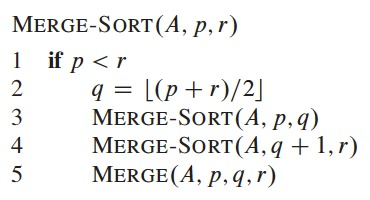# Designing Algorithms

• How Merge works: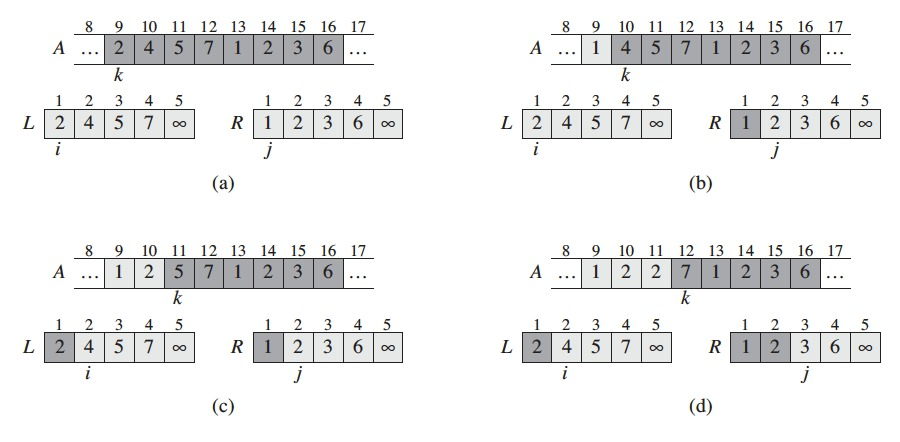# Designing Algorithms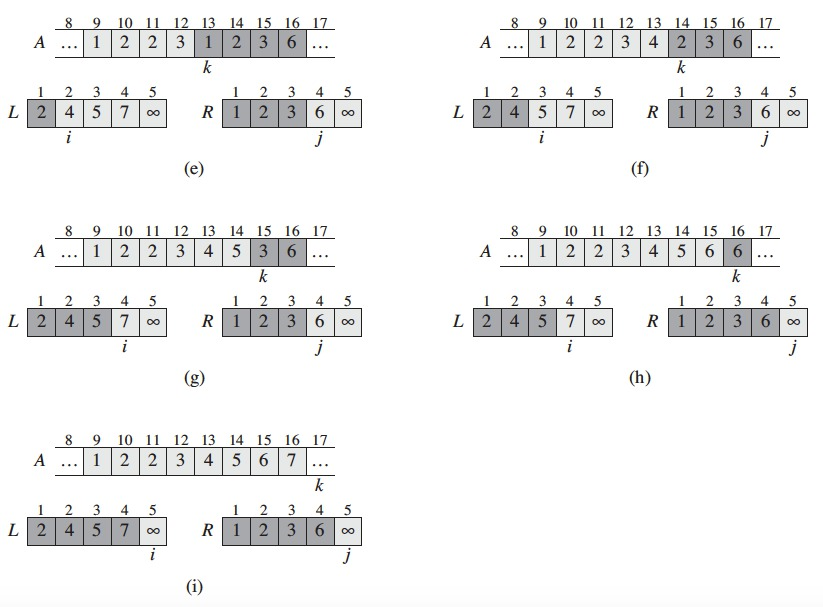# Designing Algorithms

• Recursive algorithm
• Its execution time is described with a recurrence equation
• Describes the execution time of a problem of size $$n$$ in terms of the execution time of smaller inputs We use mathematical tools to solve recurrences

# Designing Algorithms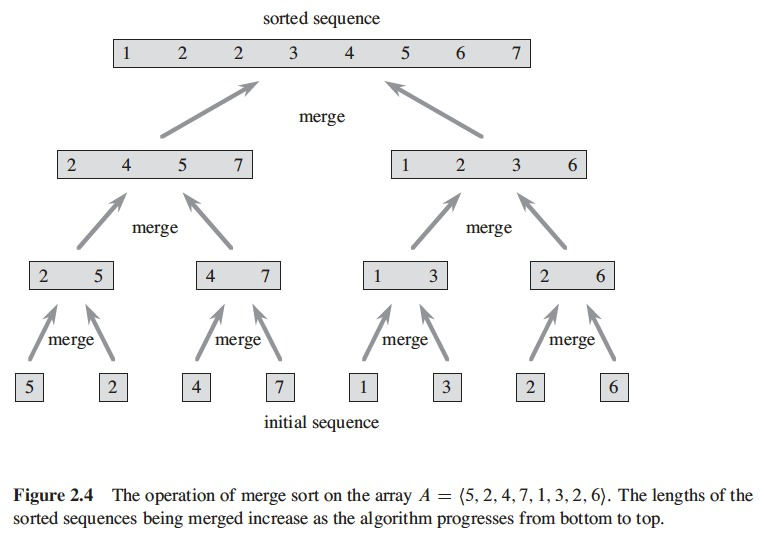# Designing Algorithms

• A recurrence for running time of a divide-and-conquer algorithm
• Comes from the 3 steps of the basic paradigm
• Let $$T(n)$$ be the running time of a problem of size $$n$$
• If problem enough small ($$n$$ < $$c$$), it takes constant time: $$\theta(1)$$

# Designing Algorithms

• A recurrence for running time of a divide-and-conquer algorithm
• Each problem division has $$a$$ subproblems, each $$1/b$$ of the original size, in MergeSort $$a = b = 2$$
• It takes $$T(n/b)$$ time to solve a subproblem of size $$n/b$$
• It takes $$aT(n/b)$$ to solve $$a$$ of them
• $$D(n)$$ is the time to divide the problem into subproblems
• $$C(n)$$ is the time to combine the solutions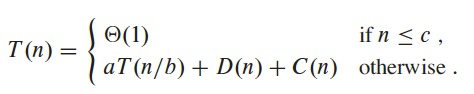# Designing Algorithms

• Analysis of MergeSort
• We assume problem size as a power of 2
• Each problem division generates 2 subsequences of size $$n/2$$

# Designing Algorithms

• Analysis of MergeSort
• Worst time execution time for MergeSort with $$n$$ numbers
• MergeSort with only one number $$\rightarrow$$ Constant time
• For $$n > 1$$ we divide the problem:
• Divide $$\rightarrow$$ Computes the middle of subarray in constant time, $$D(n) = \theta(1)$$
• Conquer: Recursively solve 2 subproblems, each of size $$n/2$$, contributes $$2T(n/2)$$ to the running time
• Combine: Merge on $$n$$-element subarray takes $$\theta(n)$$, then $$C(n) = \theta(n)$$

# Designing Algorithms

• Analysis of MergeSort
• We sum functions $$D(n)$$ and $$C(n)$$ for the analysis
• Sum $$\theta(n)$$ and $$\theta(1)$$
• We add the term $$2T(n/2)$$ to get the worst case for MergeSort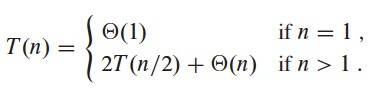• Solution for recurrence: $$T(n)$$ is $$\theta(n \lg n)$$
• For large enough inputs, MergeSort with $$\theta(n \lg n)$$ is better than InsertionSort with $$\theta(n^2)$$

# Designing Algorithms

• Solving the recurrence: $$T(n)$$ is $$\theta(n \lg n)$$
• For convenience assume that $$n$$ is an exact power of 2
• Level $$i$$ below the top has $$2^i$$ nodes, each contributing a cost of $$c(n/2^i)$$
• The $$i^{th}$$ level below the top has total cost of $$2^i c(n/2^i) = cn$$
• Bottom level has $$n$$ nodes, each contributing a cost of $$c$$, for a total of $$cn$$
• Total number of levels of the recursion tree is $$\lg n + 1$$, where $$n$$ is the number of leaves
• Corresponds to the input size
• To compute the cost, we add the costs of all levels
• There are $$\lg n + 1$$ levels, each with cost $$cn$$, for a total cost of $$cn(lgn + 1) = cn \lg n + cn$$
• We ignore the low-order term and constant $$c$$ to get $$\Theta(n \lg n)$$

# Designing Algorithms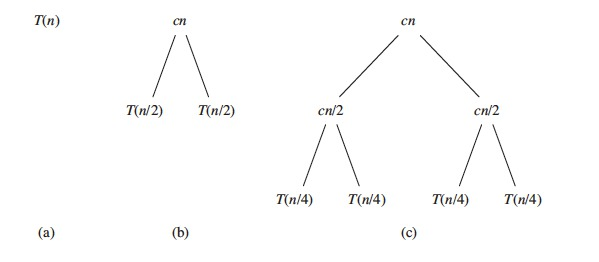# Designing Algorithms